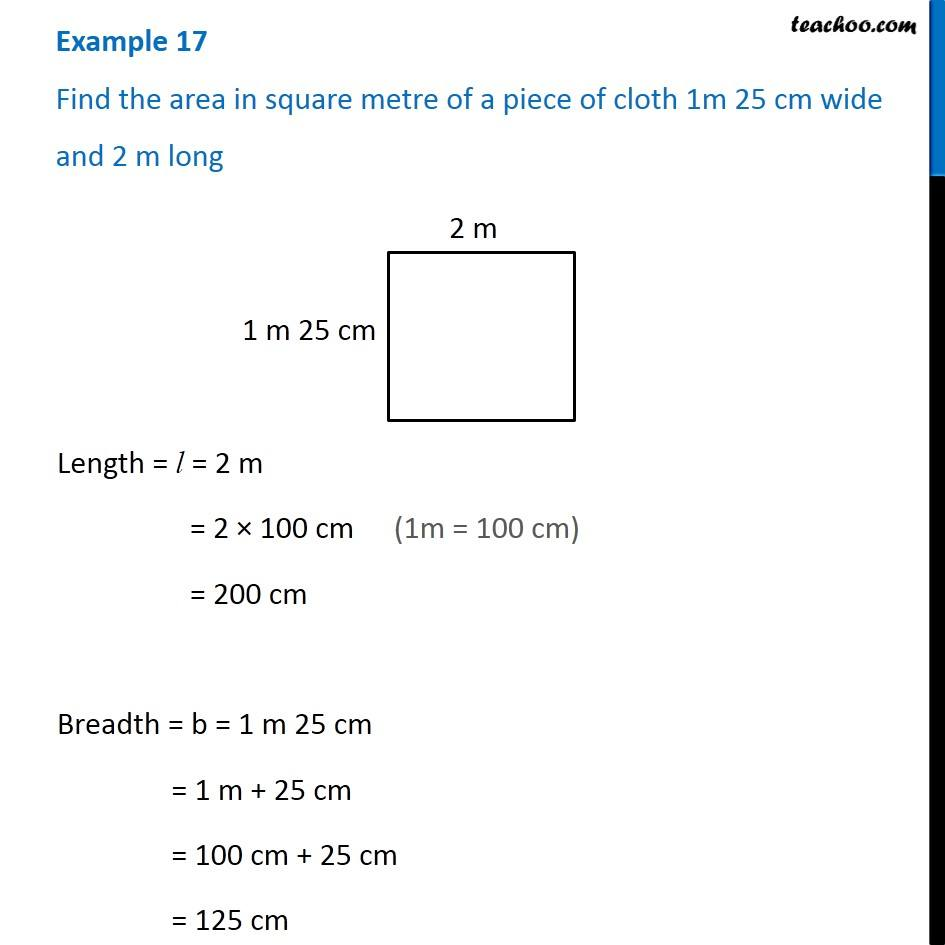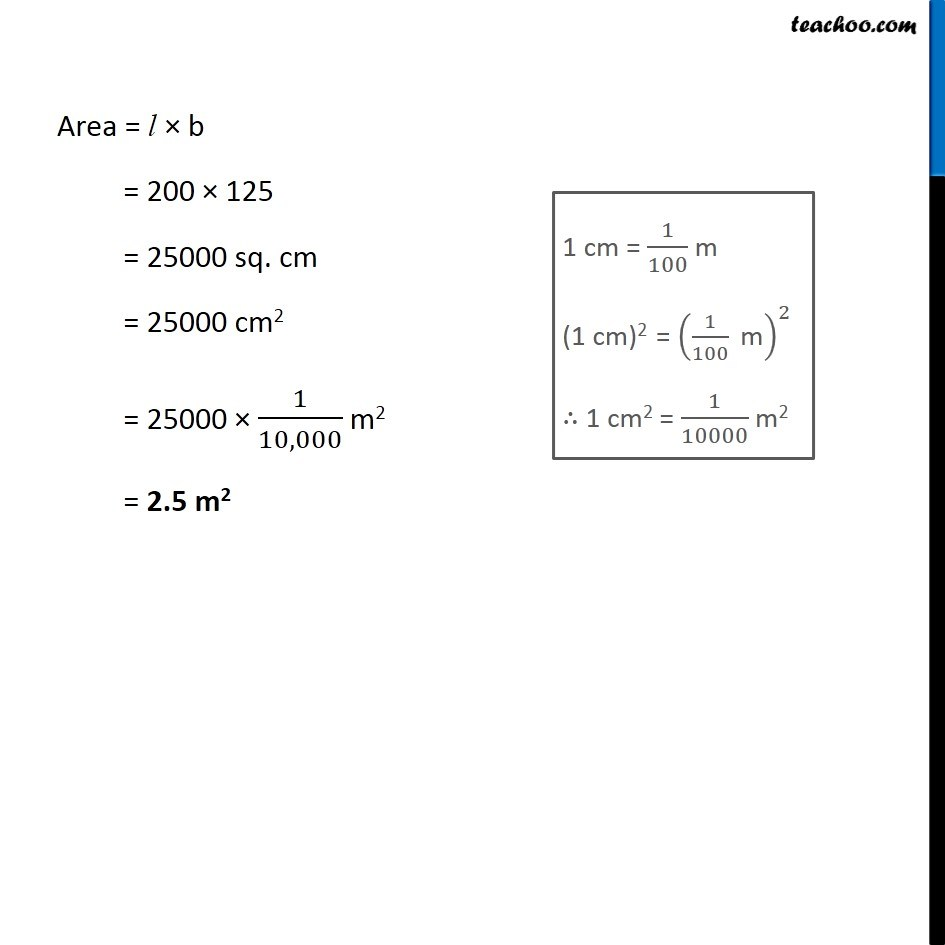Examples

Chapter 10 Class 6 Mensuration
Serial order wiseLearn in your speed, with individual attention - Teachoo Maths 1-on-1 Class

### Transcript

Example 17 Find the area in square metre of a piece of cloth 1m 25 cm wide and 2 m long Length = l = 2 m = 2 × 100 cm = 200 cm Breadth = b = 1 m 25 cm = 1 m + 25 cm = 100 cm + 25 cm = 125 cm (1m = 100 cm) Area = l × b = 200 × 125 = 25000 sq. cm = 25000 cm2 = 25000 × 1/10,000 m2 = 2.5 m2 1 cm = 1/100 m (1 cm)2 = (1/100 " m" )^2 ∴ 1 cm2 = 1/10000 m2